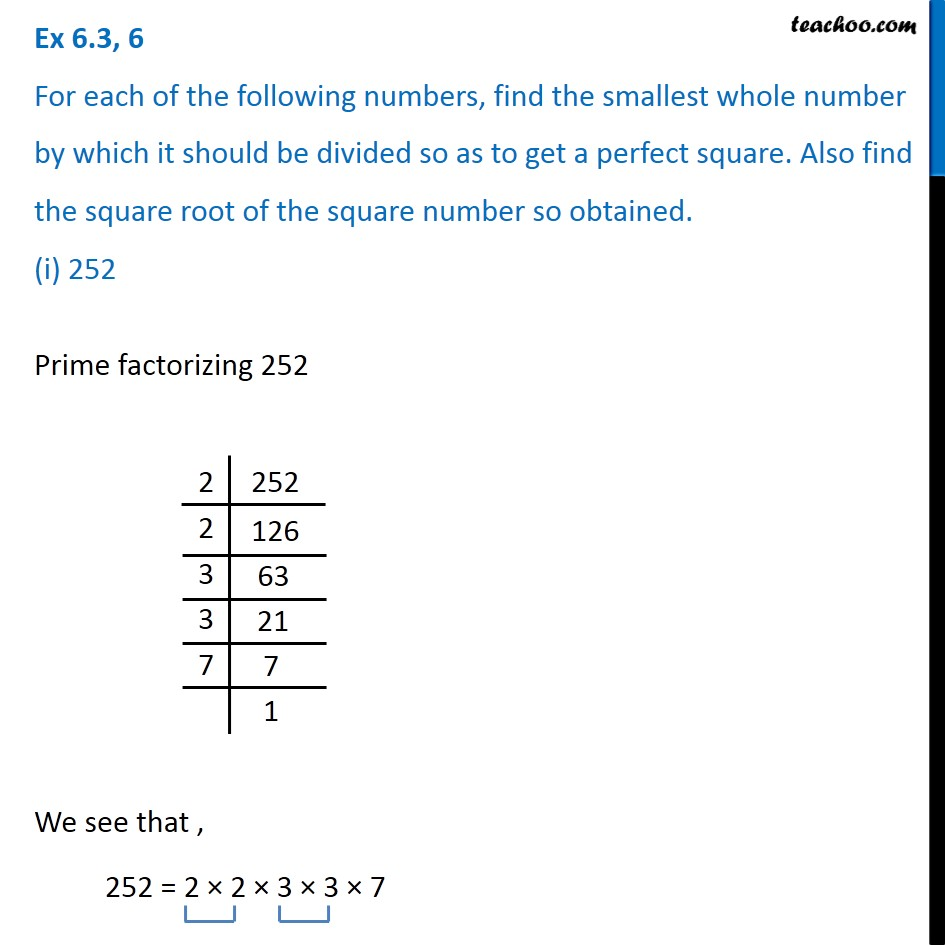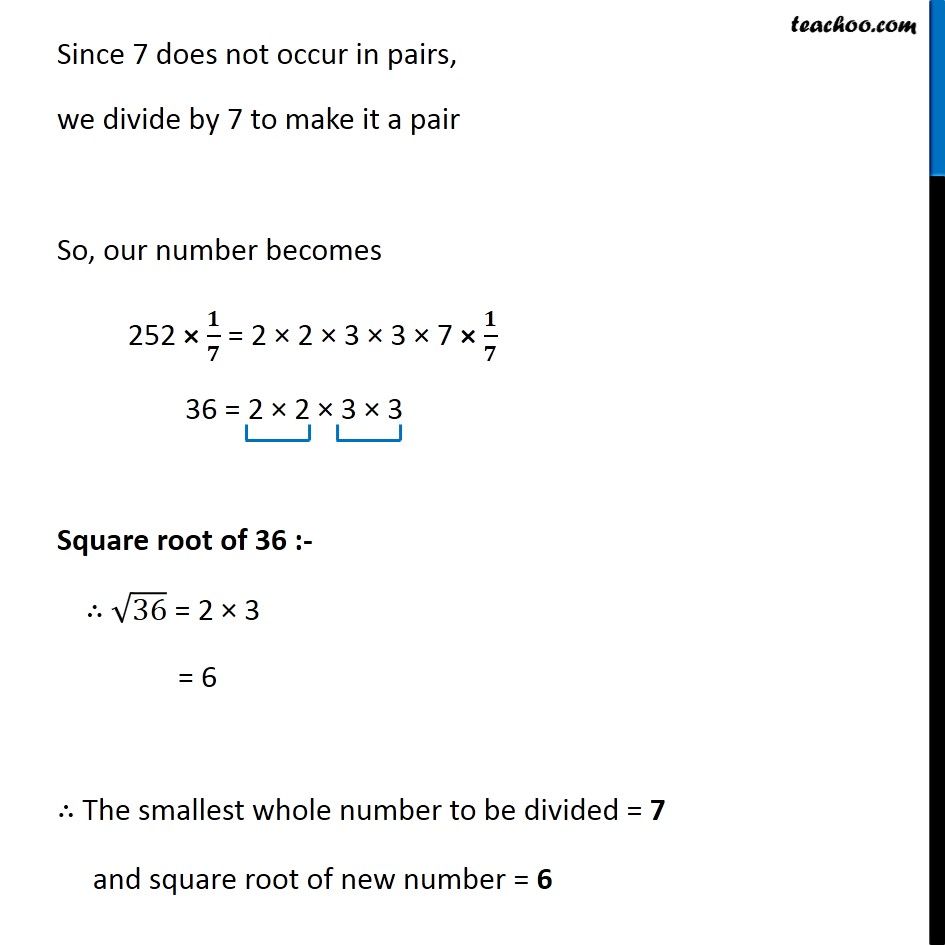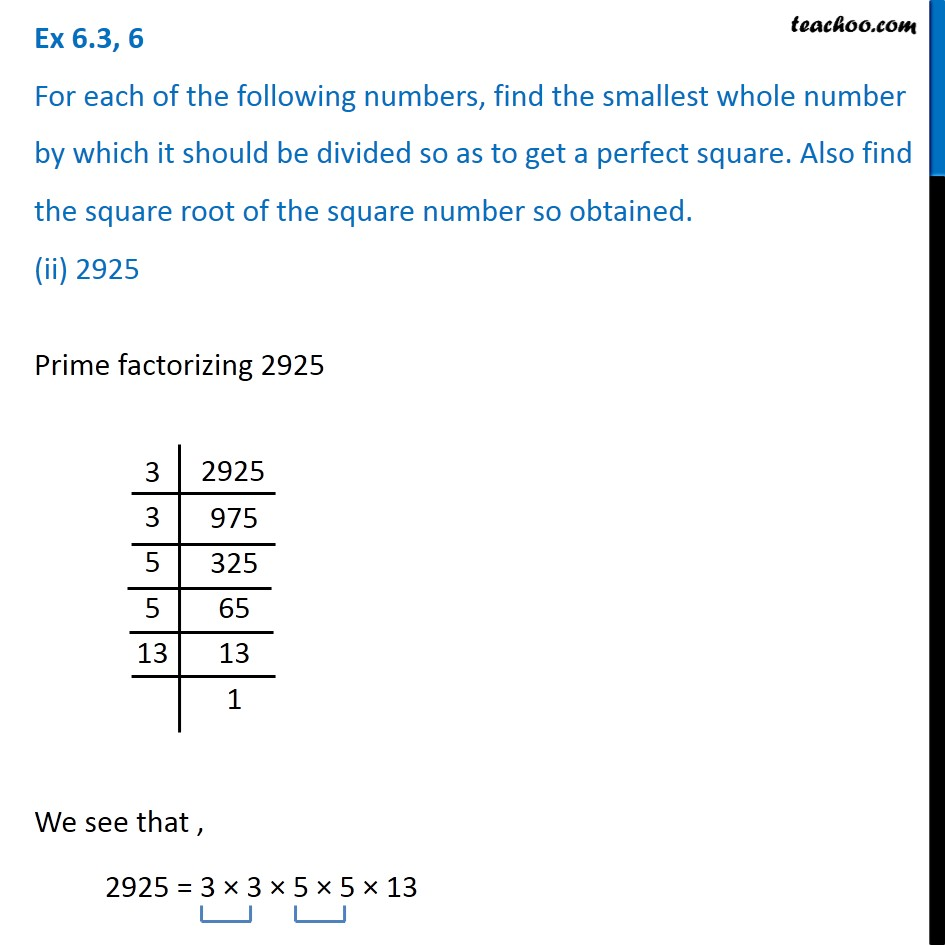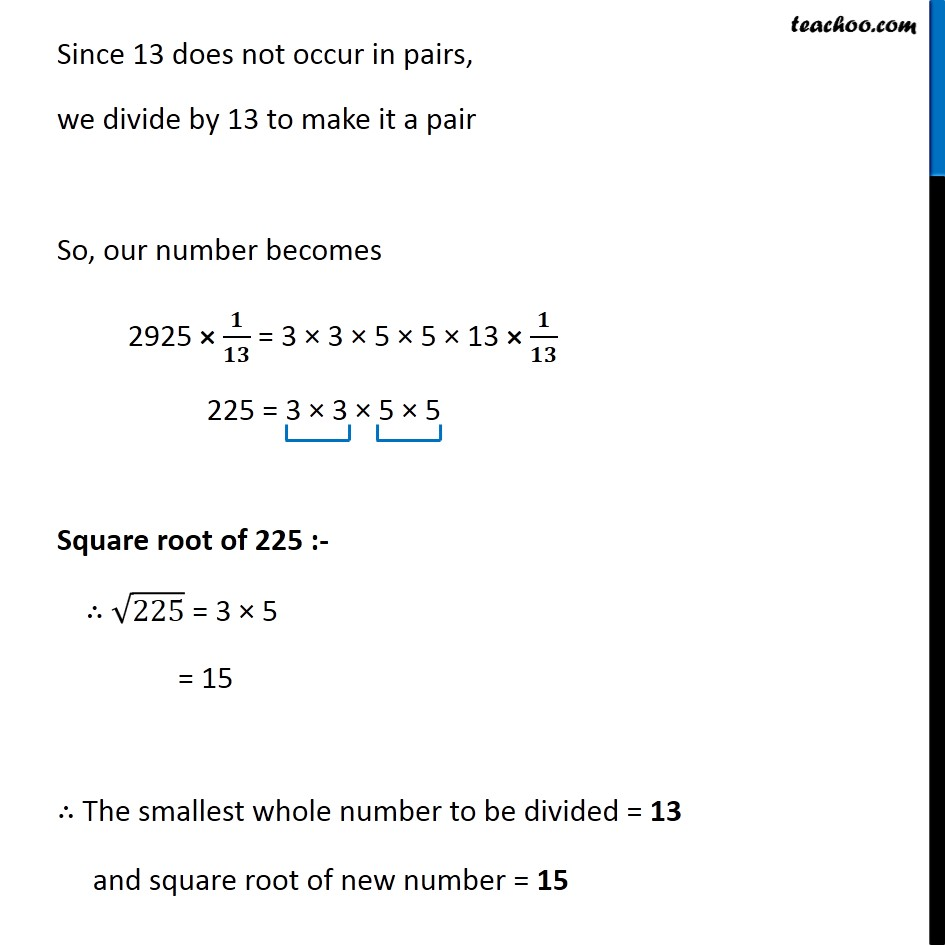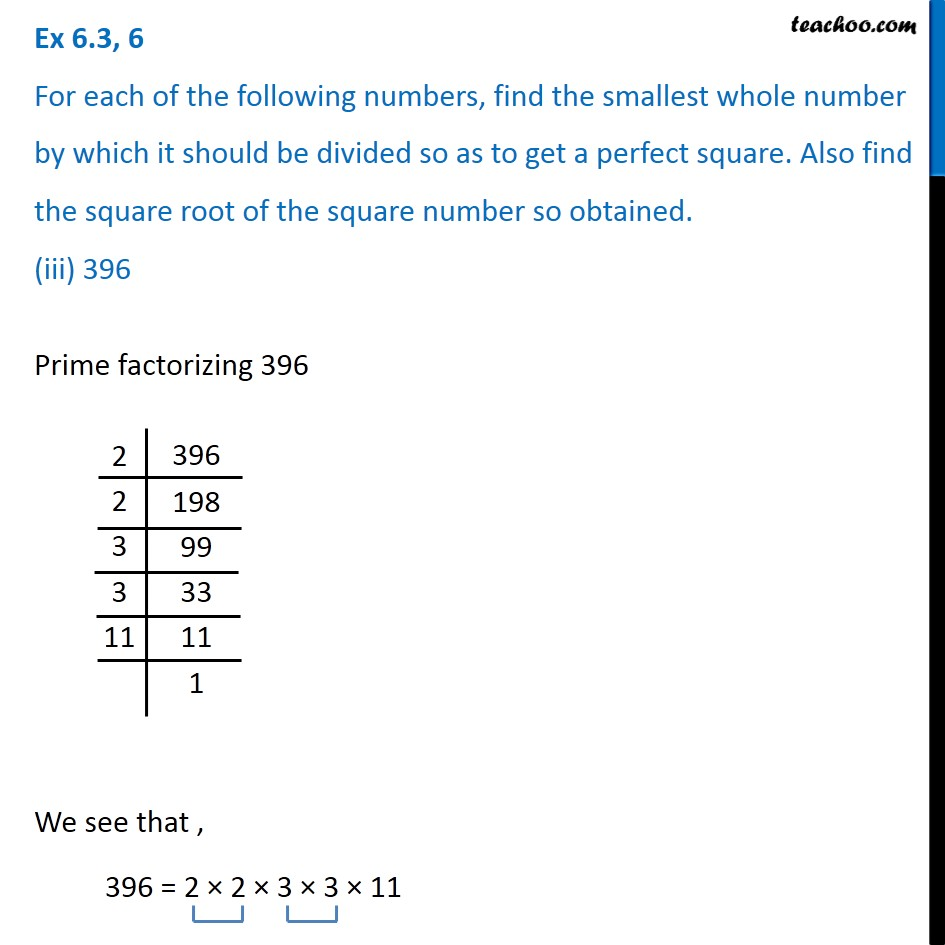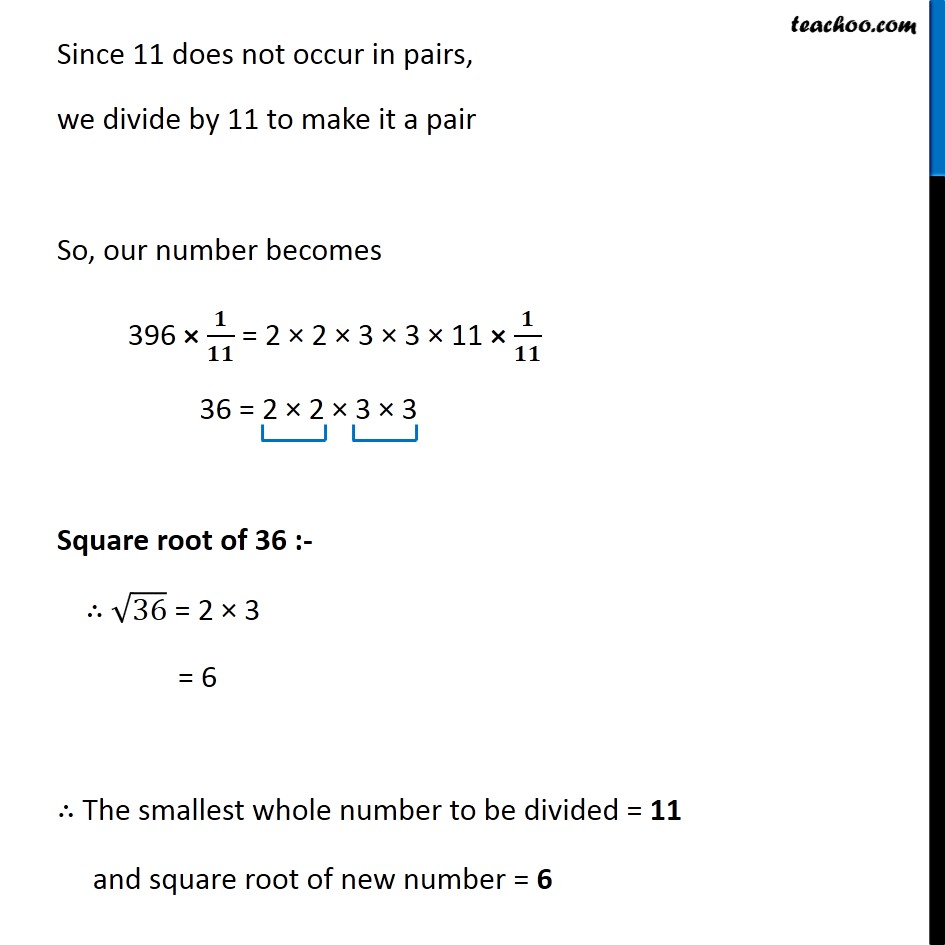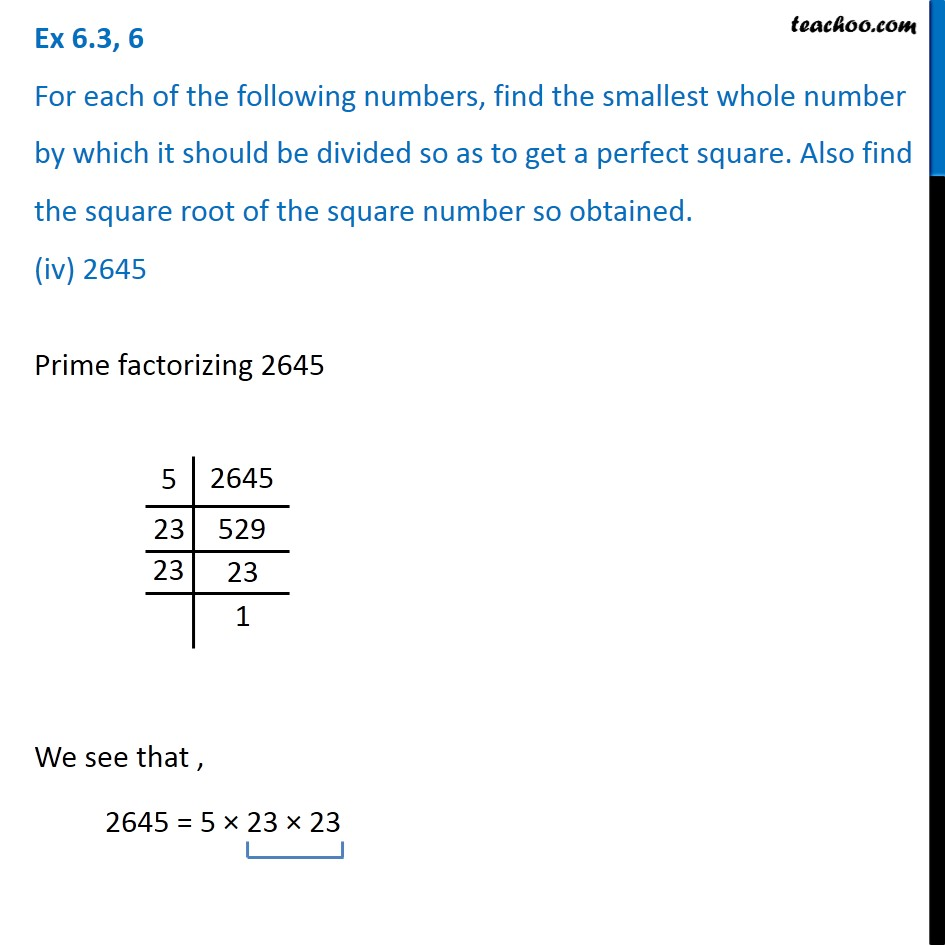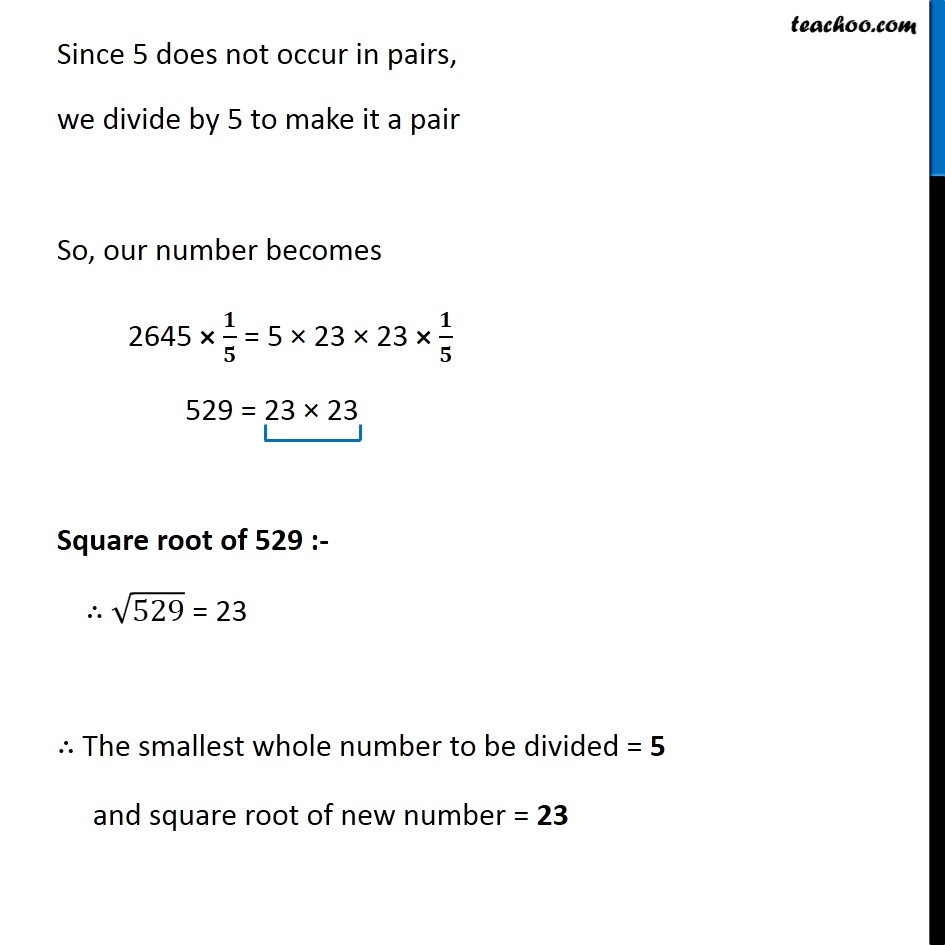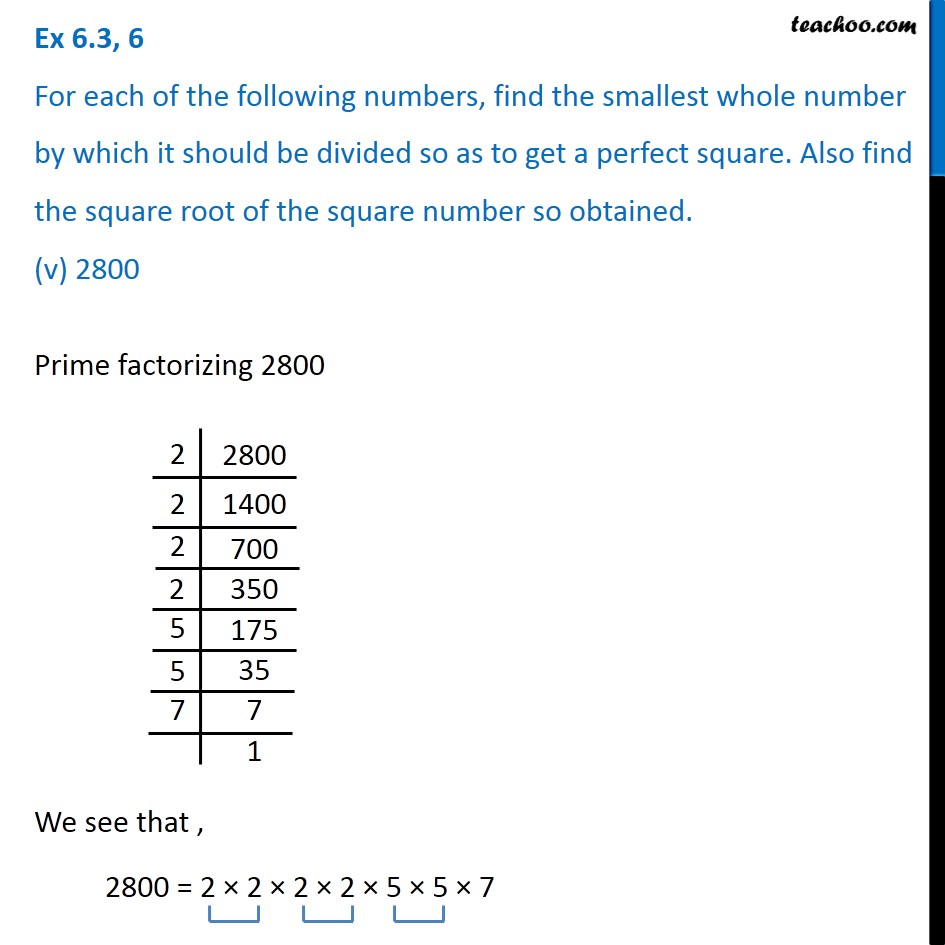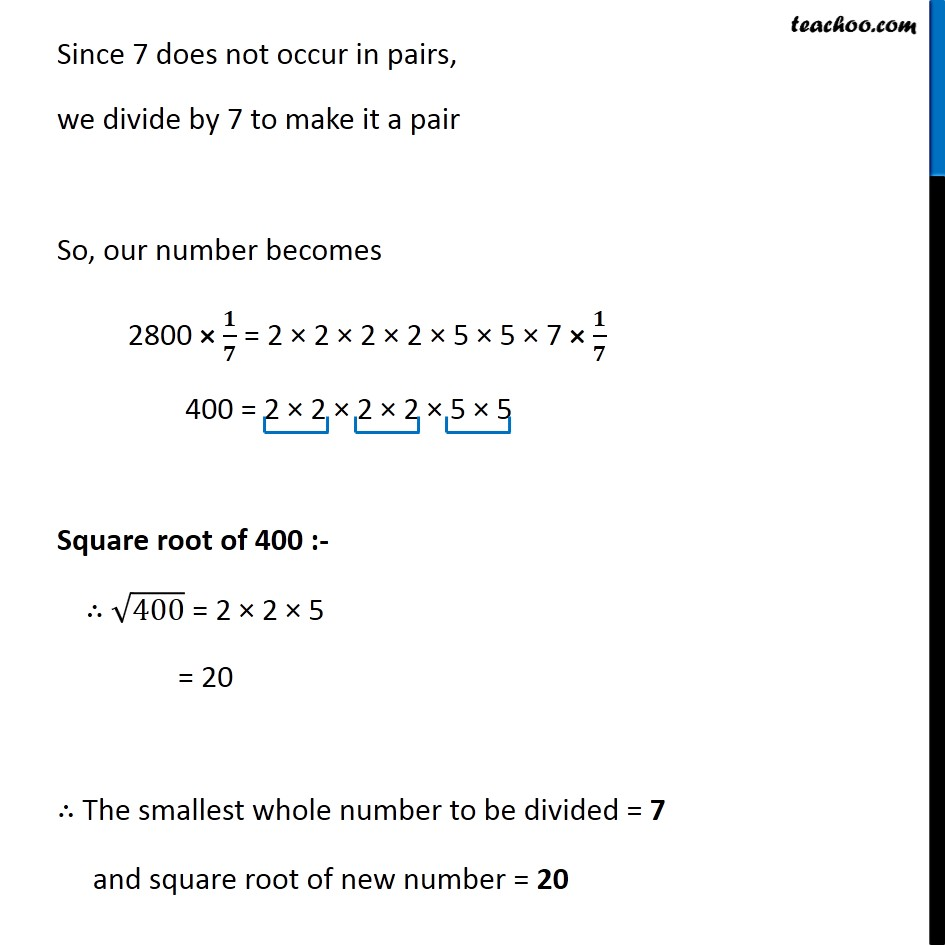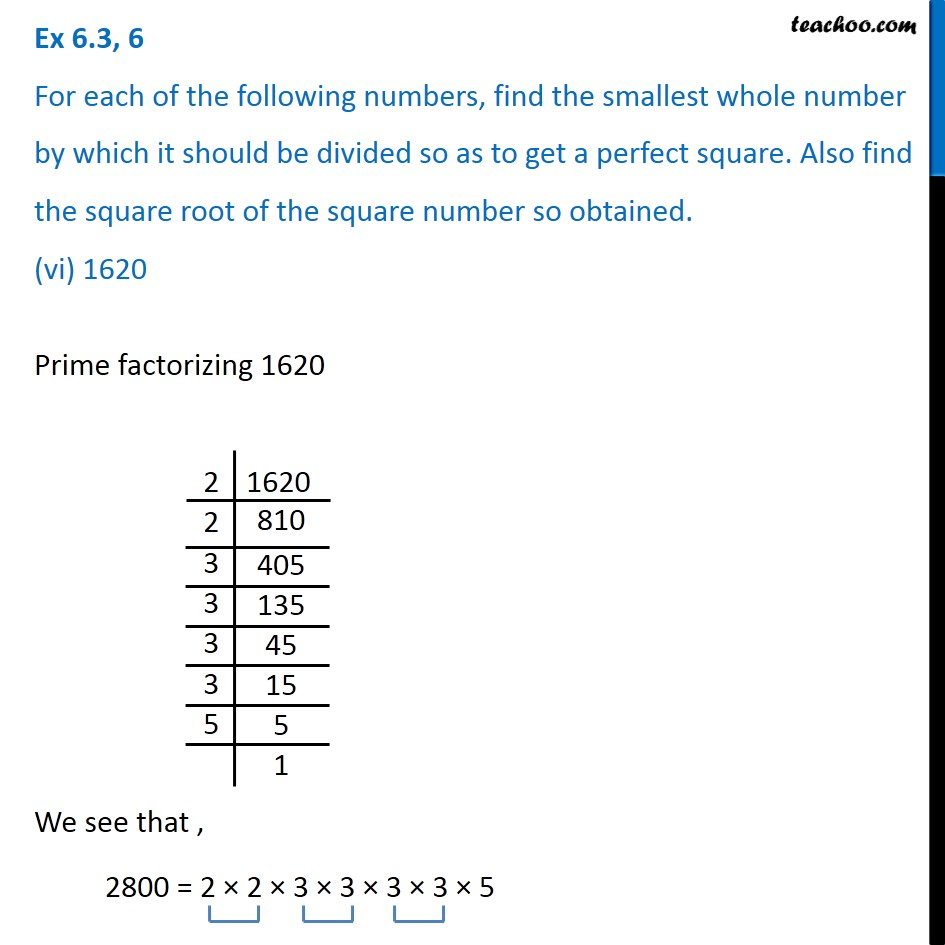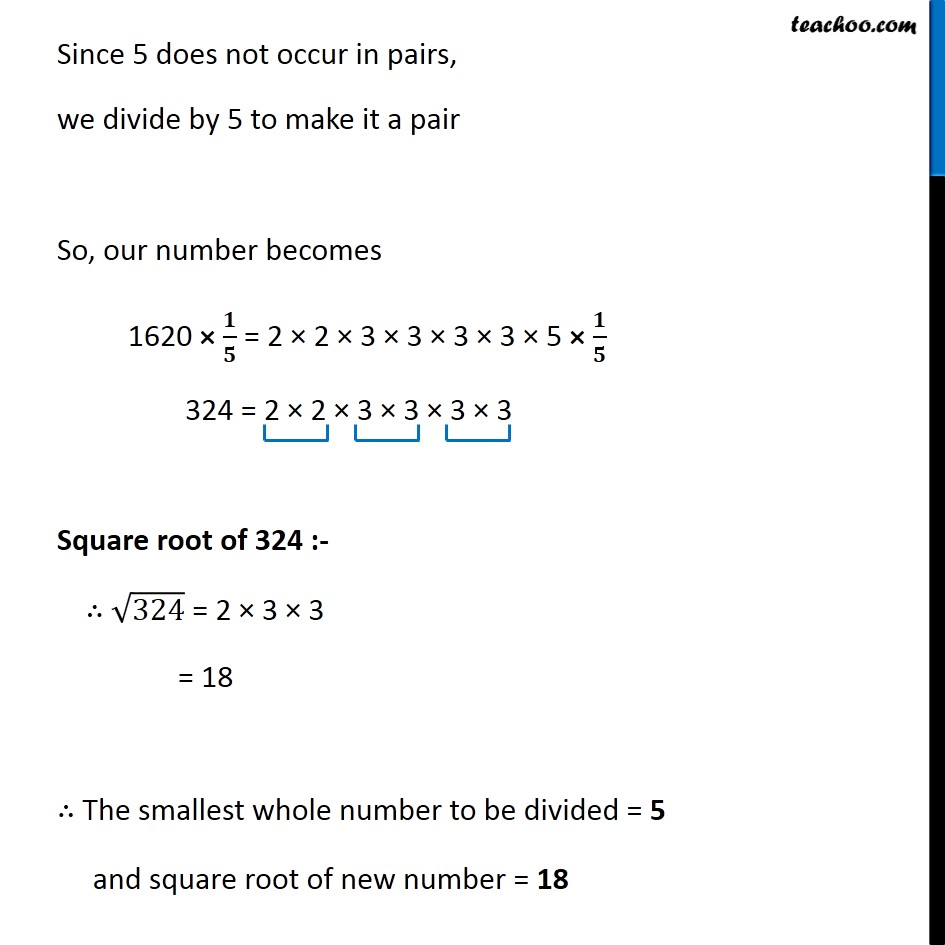Subscribe to our Youtube Channel - https://you.tube/teachoo

1. Chapter 6 Class 8 Squares and Square Roots
2. Serial order wise
3. Ex 6.3

Transcript

Ex 6.3, 6 For each of the following numbers, find the smallest whole number by which it should be divided so as to get a perfect square. Also find the square root of the square number so obtained. (i) 252Prime factorizing 252 We see that , 252 = 2 × 2 × 3 × 3 × 7 Since 7 does not occur in pairs, we divide by 7 to make it a pair So, our number becomes 252 × 𝟏/𝟕 = 2 × 2 × 3 × 3 × 7 × 𝟏/𝟕 36 = 2 × 2 × 3 × 3 Square root of 36 :- ∴ √36 = 2 × 3 = 6 ∴ The smallest whole number to be divided = 7 and square root of new number = 6 Ex 6.3, 6 For each of the following numbers, find the smallest whole number by which it should be divided so as to get a perfect square. Also find the square root of the square number so obtained. (ii) 2925Prime factorizing 2925 We see that , 2925 = 3 × 3 × 5 × 5 × 13 Since 13 does not occur in pairs, we divide by 13 to make it a pair So, our number becomes 2925 × 𝟏/𝟏𝟑 = 3 × 3 × 5 × 5 × 13 × 𝟏/𝟏𝟑 225 = 3 × 3 × 5 × 5 Square root of 225 :- ∴ √225 = 3 × 5 = 15 ∴ The smallest whole number to be divided = 13 and square root of new number = 15 Ex 6.3, 6 For each of the following numbers, find the smallest whole number by which it should be divided so as to get a perfect square. Also find the square root of the square number so obtained. (iii) 396Prime factorizing 396 We see that , 396 = 2 × 2 × 3 × 3 × 11 Since 11 does not occur in pairs, we divide by 11 to make it a pair So, our number becomes 396 × 𝟏/𝟏𝟏 = 2 × 2 × 3 × 3 × 11 × 𝟏/𝟏𝟏 36 = 2 × 2 × 3 × 3 Square root of 36 :- ∴ √36 = 2 × 3 = 6 ∴ The smallest whole number to be divided = 11 and square root of new number = 6 Ex 6.3, 6 For each of the following numbers, find the smallest whole number by which it should be divided so as to get a perfect square. Also find the square root of the square number so obtained. (iv) 2645Prime factorizing 2645 We see that , 2645 = 5 × 23 × 23 Since 5 does not occur in pairs, we divide by 5 to make it a pair So, our number becomes 2645 × 𝟏/𝟓 = 5 × 23 × 23 × 𝟏/𝟓 529 = 23 × 23 Square root of 529 :- ∴ √529 = 23 ∴ The smallest whole number to be divided = 5 and square root of new number = 23 Ex 6.3, 6 For each of the following numbers, find the smallest whole number by which it should be divided so as to get a perfect square. Also find the square root of the square number so obtained. (v) 2800Prime factorizing 2800 We see that , 2800 = 2 × 2 × 2 × 2 × 5 × 5 × 7 Since 7 does not occur in pairs, we divide by 7 to make it a pair So, our number becomes 2800 × 𝟏/𝟕 = 2 × 2 × 2 × 2 × 5 × 5 × 7 × 𝟏/𝟕 400 = 2 × 2 × 2 × 2 × 5 × 5 Square root of 400 :- ∴ √400 = 2 × 2 × 5 = 20 ∴ The smallest whole number to be divided = 7 and square root of new number = 20 Ex 6.3, 6 For each of the following numbers, find the smallest whole number by which it should be divided so as to get a perfect square. Also find the square root of the square number so obtained. (vi) 1620Prime factorizing 1620 We see that , 2800 = 2 × 2 × 3 × 3 × 3 × 3 × 5 Since 5 does not occur in pairs, we divide by 5 to make it a pair So, our number becomes 1620 × 𝟏/𝟓 = 2 × 2 × 3 × 3 × 3 × 3 × 5 × 𝟏/𝟓 324 = 2 × 2 × 3 × 3 × 3 × 3 Square root of 324 :- ∴ √324 = 2 × 3 × 3 = 18 ∴ The smallest whole number to be divided = 5 and square root of new number = 18

Ex 6.3